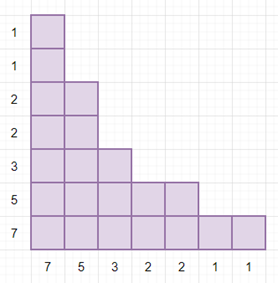# Program to check whether given list of blocks are symmetric over x = y line or not in python

Suppose we have a list of numbers called nums. And it is representing the height of square blocks, we have to check whether the shape is symmetric over the y = x line or not.

So, if the input is like nums = [7, 5, 3, 2, 2, 1, 1], then the output will be TrueTo solve this, we will follow these steps:

• i := 0
• j := size of nums - 1
• while i <= j, do
• h := nums[j]
• while i < h, do
• if nums[i] is not same as (j + 1), then
• return False
• i := i + 1
• j := j - 1
• return True

Let us see the following implementation to get better understanding:

## Example

Live Demo

class Solution:
def solve(self, nums):
i = 0
j = len(nums) - 1
while i <= j:
h = nums[j]
while i < h:
if nums[i] != j + 1:
return False
i += 1
j -= 1
return True

ob = Solution()
nums = [7, 5, 3, 2, 2, 1, 1]
print(ob.solve(nums))

## Input

[7, 5, 3, 2, 2, 1, 1]

## Output

True